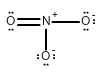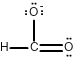# Homework 95

$$\newcommand{\vecs}{\overset { \rightharpoonup} {\mathbf{#1}} }$$ $$\newcommand{\vecd}{\overset{-\!-\!\rightharpoonup}{\vphantom{a}\smash {#1}}}$$$$\newcommand{\id}{\mathrm{id}}$$ $$\newcommand{\Span}{\mathrm{span}}$$ $$\newcommand{\kernel}{\mathrm{null}\,}$$ $$\newcommand{\range}{\mathrm{range}\,}$$ $$\newcommand{\RealPart}{\mathrm{Re}}$$ $$\newcommand{\ImaginaryPart}{\mathrm{Im}}$$ $$\newcommand{\Argument}{\mathrm{Arg}}$$ $$\newcommand{\norm}{\| #1 \|}$$ $$\newcommand{\inner}{\langle #1, #2 \rangle}$$ $$\newcommand{\Span}{\mathrm{span}}$$ $$\newcommand{\id}{\mathrm{id}}$$ $$\newcommand{\Span}{\mathrm{span}}$$ $$\newcommand{\kernel}{\mathrm{null}\,}$$ $$\newcommand{\range}{\mathrm{range}\,}$$ $$\newcommand{\RealPart}{\mathrm{Re}}$$ $$\newcommand{\ImaginaryPart}{\mathrm{Im}}$$ $$\newcommand{\Argument}{\mathrm{Arg}}$$ $$\newcommand{\norm}{\| #1 \|}$$ $$\newcommand{\inner}{\langle #1, #2 \rangle}$$ $$\newcommand{\Span}{\mathrm{span}}$$

## 8.41

Photosynthesis is a chemical reaction in plants used to produce oxygen and glucose. If a plant uses 6.3g CO2, how much water (in grams) is needed to complete the reaction? How much glucose will be formed?

$6CO_{2}+6H_{2}O+light\rightarrow C_{6}H_{12}O_{6}+6O_{2}$

Solution/ part 1: To solve this you must first convert grams of CO2 to moles of CO2. From there you cross the mole bridge, which is the mole ratio between the molecules, this simply means that you will be going from moles of one compound to moles of a different compound. Finally, you must convert the moles of H20 to grams of H20. Then, you will plug all of those numbers into your calculator to get your solution.

Step 1- The first step to solving this problem is to convert the grams of CO2 to moles of CO2. To do this you will divide 1 mol CO2 by the molar mass of CO2. You find the molar mass by looking on the periodic table. You then multiply 6.3g CO2 by the value you found which is $\frac{1molCO_{2}}{44gCO_{2}}.$

$6.3gCO_{2}\frac{1molCO_{2}}{44gCO_{2}}$

Step 2- Next, you need to cross the mole bridge. This is where you show the mole ratio between the molecules. This simply means that you will be going from moles of CO2 to moles of H20. You do this because you ae trying to solve for how much water is needed to complete the reaction and you started with CO2. The mole ratio of CO2 to H20 is 6:6. If you look at the equation you will see that. You need to divide the moles H20 by moles of CO2. You will take step 1 and multiply it with what you have found here which is $\frac{6molH_{2}O}{6molCO_{2}}$. This will give you

$6.3gCO_{2}*\frac{1molCO_{2}}{44gCO_{2}}*\frac{6molH_{2}O}{6molCO_{2}}$

Step 3- Now you need to convert mol H20 to grams of H20. To do that you need to multiply by the molar mass of H20. Once again, you can find the molar mass on the periodic table. The molar mass of H20 is 18 grams per mole.$\frac{18gH_{2}O}{1molH_{2}O}$. Now that you have this you will this value and multiply it by what you found in step 2. This will give you

$6.3gCO_{2}*\frac{1molCO_{2}}{44gCO_{2}}*\frac{6molH_{2}O}{6molCO_{2}}*\frac{18gH_{2}O}{1molH_{2}O}$

Step 4:

Now that you have all your values you just plug them into your calculator and get a value of 2.5773g H20. Since you have 2 sig figs in the question your answer should have 2 sig figs, so you get an answer of 2.6g H2O.

Solution/ part 2: To solve this part you must convert the grams of CO2 to moles of CO2. From there you cross the mole bridge, which is the mole ratio between the molecules. Finally you must convert the moles of glucose to grams of glucose. Then you will plug all of those numbers into your calculator to get your solution.

For this part you are trying to solve for how much glucose will be formed given 6.3g CO2.

Step 1- The first step to solving this problem is to convert the grams of CO2 to moles of CO2. To do this you will divide 1 mol CO2 by the molar mass of CO2. You find the molar mass by looking on the periodic table. You then multiply 6.3g CO2 by the value you found which is $\frac{1molCO_{2}}{44gCO_{2}}.$

$6.3gCO_{2}\frac{1molCO_{2}}{44gCO_{2}}$

Step 2- Next, you need to cross the mole bridge. This is where you show the mole ratio between the molecules. This simply means that you will be going from moles of CO2 to moles of C6H12O6. You do this because you ae trying to solve for how much glucose will be formed with the reaction and you started with CO2.The mole ratio of CO2 to C6H12O6 is 6:1. This is given in the equation. Now you need to divide moles of C6H12O6 by the moles of CO2. Which is $\frac{1molC_{6}H_{12}O_{6}}{6molCO_{2}}$. Then you take Step 1 and multiply is by this value to give you

$6.3gCO_{2}\frac{1molCO_{2}}{44gCO_{2}}*\frac{1molC_{6}H_{12}O_{6}}{6molCO_{2}}$

Step 3- Now you need to convert moles to grams. To do that you need to multiply by the molar mass of C6H12O6 which is 180 grams per mole.$\frac{180gC_{6}H_{12}O_{6}}{1molC_{6}H_{12}O_{6}}$. Then you multiply this value by step 2 to give you

$6.3gCO_{2}\frac{1molCO_{2}}{44gCO_{2}}*\frac{1molC_{6}H_{12}O_{6}}{6molCO_{2}}*\frac{180gC_{6}H_{12}O_{6}}{1molC_{6}H_{12}O_{6}}$

Step 4- Now that you have all your values you just plug them into your calculator and get a value of 4.295g C6H12O6. Since you had two sig figs in the question, there will be two sig figs. So your answer will be 4.2g C6H12O6 formed.

## 6.35

Draw all of the possible Lewis structures for the following ions or molecules: include resonance structures and label formal charges on each ion.

1. nitrate ion (NO3-)
2. formate ion (CHO2-)
4. ozone (O3)

The octet rule states that each atom needs to have 8 electrons. Each lone pair counts as 2. A bond also counts as 2 for each atom in the bond. Then you have to look at how many bonds each element likes to have. That is based on their column or period on the periodic table. Carbon likes to have 4 bonds, Oxygen prefers 2, Nitrogen prefers 3, and Chlorine prefers 1. If they have more or less then that it gives them a formal charge. The formula for formal charge is the [number of valance electrons] -[electrons in lone bonding pairs+1/2 the number of bonding electrons]. The Lewis structures will be as follows:

1.)<--><-->2.)<-->3.)<-->4.)<-->Homework 95 is shared under a not declared license and was authored, remixed, and/or curated by LibreTexts.